## Given the reaction: N2 + O2 = 2NO for which the Keq at 2273 K is 1.2 x 10-4 a. Write the equilibrium constant expression for the react

Question

Given the reaction: N2 + O2 = 2NO for which the Keq at 2273 K is 1.2 x 10-4
a. Write the equilibrium constant expression for the reaction.
b. Write the equation that would allow you solve for the concentration of NO.
c. What is the concentration of NO if [NZ] = 0.166M and  = 0.145M?

in progress 0
2 months 2021-07-30T10:37:29+00:00 1 Answers 3 views 0

(a): The expression of equilibrium constant is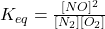(b): The equation to solve the concentration of NO is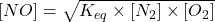(c): The concentration of NO is 0.0017 M.

Explanation:

The equilibrium constant is defined as the ratio of the concentration of products to the concentration of reactants raised to the power of the stoichiometric coefficient of each. It is represented by the term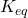(a):

The given chemical equation follows: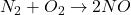The expression for equilbrium constant will be:(b):

The equation to solve the concentration of NO follows:……(1)

(c):

Given values: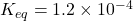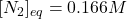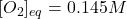Plugging values in equation 1, we get: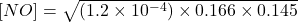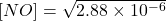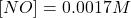Hence, the concentration of NO is 0.0017 M.